Updating search results...

# 148 Results

View
Selected filters:
• Functions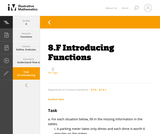Unrestricted Use
CC BY
Rating
0.0 stars

This is a task from the Illustrative Mathematics website that is one part of a complete illustration of the standard to which it is aligned. Each task has at least one solution and some commentary that addresses important aspects of the task and its potential use.

Subject:
Functions
Material Type:
Activity/Lab
Provider:
Illustrative Mathematics
Provider Set:
Illustrative Mathematics
Author:
Illustrative Mathematics
08/06/2015Unrestricted Use
CC BY
Rating
0.0 stars

The problem presents a context where a quadratic function arises. Careful analysis, including graphing, of the function is closely related to the context. The student will gain valuable experience applying the quadratic formula and the exercise also gives a possible implementation of completing the square.

Subject:
Mathematics
Functions
Material Type:
Activity/Lab
Provider:
Illustrative Mathematics
Provider Set:
Illustrative Mathematics
Author:
Illustrative Mathematics
05/01/2012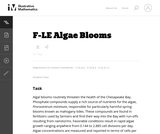Unrestricted Use
CC BY
Rating
0.0 stars

The problem statement describes a changing algae population as reported by the Maryland Department of Natural Resources. In part (a), students are expected to build an exponential function modeling algae concentration from the description given of the relationship between concentrations in cells/ml and days of rapid growth (F-LE.2).

Subject:
Mathematics
Functions
Material Type:
Activity/Lab
Provider:
Illustrative Mathematics
Provider Set:
Illustrative Mathematics
Author:
Illustrative Mathematics
05/01/2012Educational Use
Rating
0.0 stars

In this video segment from Cyberchase, Matt tries for a second time to arrange tables and chairs to accommodate 20 workers.

Subject:
Mathematics
Algebra
Functions
Material Type:
Lecture
Provider:
PBS LearningMedia
Provider Set:
PBS Learning Media: Multimedia Resources for the Classroom and Professional Development
Author:
U.S. Department of Education
WNET
09/23/2008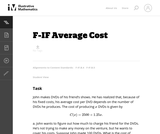Unrestricted Use
CC BY
Rating
0.0 stars

In this real world problem students solve questions based on the relationship between production costs and price.

Subject:
Mathematics
Functions
Material Type:
Activity/Lab
Provider:
Illustrative Mathematics
Provider Set:
Illustrative Mathematics
Author:
Illustrative Mathematics
05/01/2012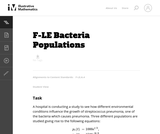Unrestricted Use
CC BY
Rating
0.0 stars

This task provides a real world context for interpreting and solving exponential equations. There are two solutions provided for part (a). The first solution demonstrates how to deduce the conclusion by thinking in terms of the functions and their rates of change. The second approach illustrates a rigorous algebraic demonstration that the two populations can never be equal.

Subject:
Mathematics
Functions
Material Type:
Activity/Lab
Provider:
Illustrative Mathematics
Provider Set:
Illustrative Mathematics
Author:
Illustrative Mathematics
05/01/2012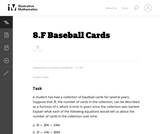Unrestricted Use
CC BY
Rating
0.0 stars

This task could be put to good use in an instructional sequence designed to develop knowledge related to students' understanding of linear functions in contexts. Though students could work independently on the task, collaboration with peers is more likely to result in the exploration of a range of interpretations.

Subject:
Mathematics
Functions
Material Type:
Activity/Lab
Provider:
Illustrative Mathematics
Provider Set:
Illustrative Mathematics
Author:
Illustrative Mathematics
05/01/2012Unrestricted Use
CC BY
Rating
0.0 stars

This task involves a fairly straightforward decaying exponential. Filling out the table and developing the general formula is complicated only by the need to work with a fraction that requires decisions about rounding and precision.

Subject:
Mathematics
Functions
Material Type:
Activity/Lab
Provider:
Illustrative Mathematics
Provider Set:
Illustrative Mathematics
Author:
Illustrative Mathematics
05/01/2012Unrestricted Use
CC BY
Rating
0.0 stars

This task describes two linear functions using two different representations. To draw conclusions about the quantities, students have to find a common way of describing them. We have presented three solutions (1) Finding equations for both functions. (2) Using tables of values. (3) Using graphs.

Subject:
Mathematics
Functions
Material Type:
Activity/Lab
Provider:
Illustrative Mathematics
Provider Set:
Illustrative Mathematics
Author:
Illustrative Mathematics
05/01/2012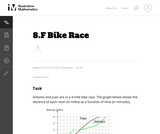Unrestricted Use
CC BY
Rating
5.0 stars

The purpose of this task is for students to interpret two distance-time graphs in terms of the context of a bicycle race. There are two major mathematical aspects to this: interpreting what a particular point on the graph means in terms of the context, and understanding that the "steepness" of the graph tells us something about how fast the bicyclists are moving.

Subject:
Mathematics
Functions
Material Type:
Activity/Lab
Provider:
Illustrative Mathematics
Provider Set:
Illustrative Mathematics
Author:
Illustrative Mathematics
05/01/2012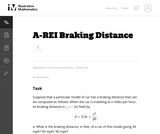Unrestricted Use
CC BY
Rating
0.0 stars

This task provides an exploration of a quadratic equation by descriptive, numerical, graphical, and algebraic techniques. Based on its real-world applicability, teachers could use the task as a way to introduce and motivate algebraic techniques like completing the square, en route to a derivation of the quadratic formula.

Subject:
Mathematics
Algebra
Functions
Material Type:
Activity/Lab
Provider:
Illustrative Mathematics
Provider Set:
Illustrative Mathematics
Author:
Illustrative Mathematics
05/01/2012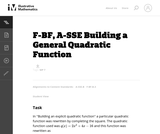Unrestricted Use
CC BY
Rating
0.0 stars

This task is for instructional purposes only and builds on ``Building an explicit quadratic function.''

Subject:
Mathematics
Algebra
Functions
Material Type:
Activity/Lab
Provider:
Illustrative Mathematics
Provider Set:
Illustrative Mathematics
Author:
Illustrative Mathematics
08/20/2012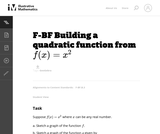Unrestricted Use
CC BY
Rating
0.0 stars

This is the first of a series of task aiming at understanding the quadratic formula in a geometric way in terms of the graph of a quadratic function.

Subject:
Mathematics
Functions
Material Type:
Activity/Lab
Provider:
Illustrative Mathematics
Provider Set:
Illustrative Mathematics
Author:
Illustrative Mathematics
08/15/2012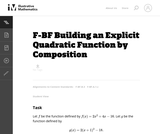Unrestricted Use
CC BY
Rating
0.0 stars

This task is intended for instruction and to motivate "Building a general quadratic function.''

Subject:
Mathematics
Functions
Material Type:
Activity/Lab
Provider:
Illustrative Mathematics
Provider Set:
Illustrative Mathematics
Author:
Illustrative Mathematics
08/26/2013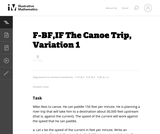Unrestricted Use
CC BY
Rating
0.0 stars

The purpose of this task is to give students practice constructing functions that represent a quantity of interest in a context, and then interpreting features of the function in the light of that context. It can be used as either an assessment or a teaching task.

Subject:
Mathematics
Functions
Material Type:
Activity/Lab
Provider:
Illustrative Mathematics
Provider Set:
Illustrative Mathematics
Author:
Illustrative Mathematics
05/01/2012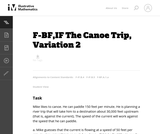Unrestricted Use
CC BY
Rating
0.0 stars

The primary purpose of this task is to lead students to a numerical and graphical understanding of the behavior of a rational function near a vertical asymptote, in terms of the expression defining the function. The canoe context focuses attention on the variables as numbers, rather than as abstract symbols.

Subject:
Mathematics
Functions
Material Type:
Activity/Lab
Provider:
Illustrative Mathematics
Provider Set:
Illustrative Mathematics
Author:
Illustrative Mathematics
05/01/2012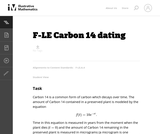Unrestricted Use
CC BY
Rating
0.0 stars

The task requires the student to use logarithms to solve an exponential equation in the realistic context of carbon dating, important in archaeology and geology, among other places. Students should be guided to recognize the use of the natural logarithm when the exponential function has the given base of e, as in this problem. Note that the purpose of this task is algebraic in nature -- closely related tasks exist which approach similar problems from numerical or graphical stances.

Subject:
Mathematics
Functions
Material Type:
Activity/Lab
Provider:
Illustrative Mathematics
Provider Set:
Illustrative Mathematics
Author:
Illustrative Mathematics
05/01/2012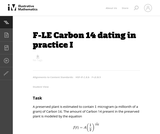Unrestricted Use
CC BY
Rating
0.0 stars

In the task "Carbon 14 Dating'' the amount of Carbon 14 in a preserved plant is studied as time passes after the plant has died. In practice, however, scientists wish to determine when the plant died and, as this task shows, this is not possible with a simple measurement of the amount of Carbon 14 remaining in the preserved plant. The equation for the amount of Carbon 14 remaining in the preserved plant is in many ways simpler here, using 12 as a base.

Subject:
Mathematics
Functions
Material Type:
Activity/Lab
Provider:
Illustrative Mathematics
Provider Set:
Illustrative Mathematics
Author:
Illustrative Mathematics
08/21/2012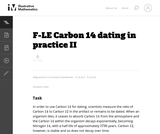Unrestricted Use
CC BY
Rating
0.0 stars

This problem introduces the method used by scientists to date certain organic material. It is based not on the amount of the Carbon 14 isotope remaining in the sample but rather on the ratio of Carbon 14 to Carbon 12. This ratio decreases, hypothetically, at a constant exponential rate as soon as the organic material has ceased to absorb Carbon 14, that is, as soon as it dies. This problem is intended for instructional purposes only. It provides an interesting and important example of mathematical modeling with an exponential function.

Subject:
Mathematics
Functions
Material Type:
Activity/Lab
Provider:
Illustrative Mathematics
Provider Set:
Illustrative Mathematics
Author:
Illustrative Mathematics
08/21/2012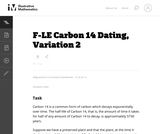Unrestricted Use
CC BY
Rating
0.0 stars

This exploratory task requires the student to use a property of exponential functions in order to estimate how much Carbon 14 remains in a preserved plant after different amounts of time.

Subject:
Mathematics
Functions
Material Type:
Activity/Lab
Provider:
Illustrative Mathematics
Provider Set:
Illustrative Mathematics
Author:
Illustrative Mathematics